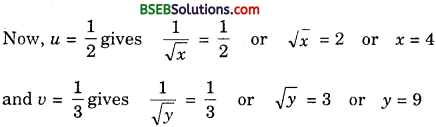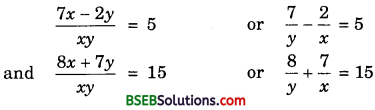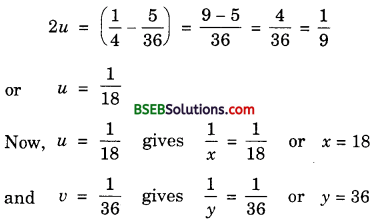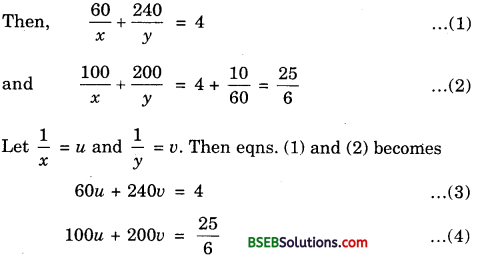# Bihar Board Class 10th Maths Solutions Chapter 3 Pair of Linear Equations in Two Variables Ex 3.6

Bihar Board Class 10th Maths Solutions Chapter 3 Pair of Linear Equations in Two Variables Ex 3.6 Textbook Questions and Answers.

## BSEB Bihar Board Class 10th Maths Solutions Chapter 3 Pair of Linear Equations in Two Variables Ex 3.6

Question 1.
Solve the following pairs of equations by reducing them to a pair of linear equations:
1. $$\frac{1}{2x}$$ + $$\frac{1}{3y}$$ = 2
$$\frac{1}{3x}$$ + $$\frac{1}{2y}$$ = $$\frac{13}{6}$$

2. $$\frac{2}{\sqrt{x}}$$ + $$\frac{3}{\sqrt{y}}$$ = 2
$$\frac{4}{\sqrt{x}}$$ – $$\frac{9}{\sqrt{y}}$$ = – 1

3. $$\frac{4}{x}$$ + 3y = 14
$$\frac{3}{x}$$ – 4y = 23

4. $$\frac{5}{x-1}$$ + $$\frac{1}{y-2}$$ = 2
$$\frac{6}{x-1}$$ – $$\frac{3}{y-2}$$ = 1

5. $$\frac{7x-2y}{xy}$$ = 5
$$\frac{8x+7y}{xy}$$ = 15

6. 6x + 3y = 6xy
2x + 4y = 5xy

7. $$\frac{10}{x+y}$$ + $$\frac{2}{x-y}$$ = 4
$$\frac{15}{x+y}$$ – $$\frac{5}{x-y}$$ = – 2

8. $$\frac{1}{3x+y}$$ + $$\frac{1}{3x-y}$$ = $$\frac{3}{4}$$
$$\frac{1}{2(3x+y)}$$ – $$\frac{1}{2(3x-y)}$$ = $$\frac{-1}{8}$$
Solution:
1. Taking $$\frac{1}{x}$$ = u and $$\frac{1}{y}$$ = v, the given system of equations becomes
$$\frac{1}{2}$$u + $$\frac{1}{3}$$v = 2 or 3u + 2v = 12 ……………… (1)
$$\frac{1}{3}$$u + $$\frac{1}{2}$$v = $$\frac{13}{6}$$ or 2u + 3v = 13 ………………. (2)
Multliplying (1) by 3 and (2) by 2, we have:
9u + 6v = 36 ………………. (3)
and 4u + 6v = 26 ………………. (4)
Substracting (4) from (3), we get
5u = 10 or u = 2
Putting u = 2 in (3), we get
18 + 6v = 36 or 6v = 18 or v = 3
Now, u = 2 gives $$\frac{1}{x}$$ = 2 or x = $$\frac{1}{2}$$
and v = 3 gives $$\frac{1}{y}$$ = 3 or y = $$\frac{1}{3}$$
Hence, the solution is x = $$\frac{1}{2}$$, y = $$\frac{1}{3}$$.

2. The given system of equation is
$$\frac{2}{\sqrt{x}}$$ + $$\frac{3}{\sqrt{y}}$$ = 2 and $$\frac{4}{\sqrt{x}}$$ – $$\frac{9}{\sqrt{y}}$$ = – 1
Putting u = $$\frac{1}{\sqrt{x}}$$ and v = $$\frac{1}{\sqrt{y}}$$, the given equations becomes
2u + 3v = 2 ………………. (1)
and 4u – 9v = – 1 ……………… (2)
6u + 9v = 6 …………………… (3)
Adding (2) and (3), we get
10u = 5 or u = $$\frac{5}{10}$$ = $$\frac{1}{2}$$
Putting u = $$\frac{1}{2}$$ in (1), we get
2 × $$\frac{1}{2}$$ + 3v = 2 or 3v = 1 or v = $$\frac{1}{3}$$Hence, the solution is x = 4, y = 9.

3. The given system of equation is
$$\frac{4}{x}$$ + 3y = 14 …………………. (1)
and $$\frac{3}{x}$$ – 4y = 23 ………………. (2)
Multliplying (1) by 4 and (2) by 3, we get
$$\frac{16}{x}$$ + 12y = 56 ……………….. (3)
and $$\frac{9}{x}$$ – 12y = 69 ……………….. (4)
Adding (3) and (4), we get
$$\frac{25}{x}$$ = 125 or x = $$\frac{25}{125}$$ = $$\frac{1}{5}$$
Putting x = $$\frac{1}{5}$$ in (1), we get
4 × 5 + 3y = 14 or 3y = 14 – 20
or 3y = – 6 or y = – 2
Hence, the solution is x = $$\frac{1}{5}$$, y = – 2.

4. Let u = $$\frac{1}{x-1}$$ and v = $$\frac{1}{y-2}$$. Then, the given system of equations becomes
5u + v = 2 …………………. (1)
and 6u – 3v = 1 ……………….. (2)
Multliplying (1) by 3, we get
15u + 3v = 6 ………………… (3)
Adding (2) and (3), we get
21u = 7 or u = $$\frac{1}{3}$$
Putting u = $$\frac{1}{3}$$ in (1), we get
$$\frac{5}{3}$$ + v = 2 or v = 2 – $$\frac{5}{3}$$ = $$\frac{6-5}{3}$$ = $$\frac{1}{3}$$
Now, u = $$\frac{1}{3}$$ gives
$$\frac{1}{x-1}$$ = $$\frac{1}{3}$$ or x – 1 = 3 or x = 4
v = $$\frac{1}{3}$$ gives
$$\frac{1}{y-2}$$ = $$\frac{1}{3}$$ or y – 2 = 3 or y = 5
Hence, the solution is x = 4, y = 5.

5. The given system of equation isLet u = $$\frac{1}{x}$$ and v = $$\frac{1}{y}$$. Then, the above equations become 7v – 2u = 5 …………… (1)
and 8v + 7u = 15 …………….. (2)
Multliplying (1) by 7 and (2) by 2, we get
49u – 14u = 35 ………………. (3)
and 16v + 14u = 30 ………………. (4)
Adding (3) and (4), we get
65v = 65 or v = 1
Putting v = 1 in (1), we get
7 – 2u = 5 or – 2u = – 2 or u = 1
Now, u = 1 gives $$\frac{1}{x}$$ = 1 or x = 1
and v = 1 gives $$\frac{1}{y}$$ = 1 or y = 1
Hence, the solution is x = 1, y = 1.

6. The given system of equations is 6x + 3y = 6xy and 2x + 4y = 5xy, where x and y are non-zero.
Since x ≠ 0, y ≠ 0, we have xy ≠ 0.
On dividing each one of the given equatìons by xy, we get
$$\frac{3}{x}$$ + $$\frac{6}{y}$$ = 6 and $$\frac{4}{x}$$ + $$\frac{2}{y}$$ = 5
Taking $$\frac{1}{x}$$ = u and $$\frac{1}{y}$$ = v, the above equations become
3u + 6v = 6 …………….. (1)
and 4u + 2v = 5 ………………. (2)
Multiplying (2) by 3, we get
12u + 6v = 15
Subtracting (1) from (3), we get
9u = 15 – 6 = 9 or u = 1
Putting u = 1 in (1), we get
3 × 1 + 6v = 6 or 6v = 6 – 3 = 3
or v = $$\frac{1}{2}$$
Now, u = 1 gives $$\frac{1}{x}$$ = 1 or x = 1
and v = $$\frac{1}{2}$$ gives $$\frac{1}{y}$$ = $$\frac{1}{2}$$ or y = 2
Hence, the given system of equations has one solution x = 1, y = 2.

7. The given system of equations is
$$\frac{10}{x+y}$$ + $$\frac{2}{x-y}$$ = 4
$$\frac{15}{x+y}$$ – $$\frac{5}{x-y}$$ = – 2
Putting u = $$\frac{1}{x+y}$$ and v = $$\frac{1}{x-y}$$, the given equations become
10u + 2v = 4 or 5u + v = 2 ………………. (1)
and 15u – 5v = – 2
Putting u = $$\frac{1}{x+y}$$ and v = $$\frac{1}{x-y}$$, the given equations become
10u + 2v = 4 or 5u + v = 2 ……………… (1)
and 15u – 5v = – 2 ………………… (2)
Multiplying (1) by 5, we get
25u + 5v = 10 ………………… (3)
Adding (2) and (3), we get
40u = 8 or u = $$\frac{1}{5}$$
Putting u = $$\frac{1}{5}$$ in (1), we get
5($$\frac{1}{5}$$) + v = 2 or v = 2 – 1 = 1
Now, u = $$\frac{1}{5}$$ gives x + y = 5 ……………….. (4)
v = 1 gives $$\frac{1}{x-y}$$ = 1
or x – y = 1 …………………. (5)Question 2.
Formulate the following problems as a pair of equations, and hence find their solutions:
1. Ritu can row downstream 20 km in 2 hours, and upstream 4 km in 2 hours. Find her speed of rowing in still water and the speed of the current.

2. 2 women and 5 men can together finish an embroidery work in 4 days, while 3 women and 6 men can finish it in 3 days. Find the time taken by 1 woman alone to finish the work, and also that taken by 1 man alone.

3. Roohi travels 300 km to her home partly by train and partly by bus. She takes 4 hours, if she travels 60 km by train and the remaining by bus.
If she travels 100 km by train and the remaining by bus, she takes 10 minutes longer. Find the speed of the train and the bus separately.
Solution:
1. Let the speed of the boat in still water be x km/h and the speed of the current be y km/h. Then,
Speed upstream = (x – y) km/h
Speed downstream = (x + y) km/h
Time taken to cover 20 km downstream
= 2 hours
or $$\frac{20}{x+y}$$ = 2 or x + y = 10 ……………….. (1)
Time taken to cover 4 km upstream = 2 hours
or $$\frac{4}{x-y}$$ = 2 or x – y = 2 ………………. (2)
Adding (1) and (2) we get,
2x = 12 or x = 6
Putting x = 6 in (1), we get
6 + y = 10 or y = 10 – 6 = 4
Hence, the speed of boat in still water = 6km/hr
and speed of current = 4km/hr

2. Let 1 woman can finish the embroidery in x days and 1 man can finish the embroidery in x days and 1 man can finish the embroidery in y days.
Then, 1 woman’s 1 day’s work = $$\frac{1}{x}$$
1 man’s 1 day’s work = $$\frac{1}{y}$$
∴ $$\frac{2}{x}$$ + $$\frac{5}{y}$$ = $$\frac{1}{4}$$ and $$\frac{3}{x}$$ + $$\frac{6}{y}$$ = $$\frac{1}{3}$$
Putting $$\frac{1}{x}$$ = u and $$\frac{1}{y}$$ = v, these equations become
2u + 5v = $$\frac{1}{4}$$ …………….. (1)
and 3v + 6v = $$\frac{1}{3}$$ ……………. (2)
Multiplying (1) by 3 and (2) by 2 and substracting, we get
3v = $$\frac{1}{12}$$ or v = $$\frac{1}{36}$$
Putting v = $$\frac{1}{36}$$ in (1), we getThus, 1 woman alone can finish the embroidery in 18 days and 1 man alone can finish it in 36 days.

3. Let the speed of train be x km/hr and speed of bus be y km/hr.Multiplying eqn. (3) by 5 and eqn. (4) by 6, we get∴ Speed of train is 60 km/hr and speed on bus is 80 km/hr.